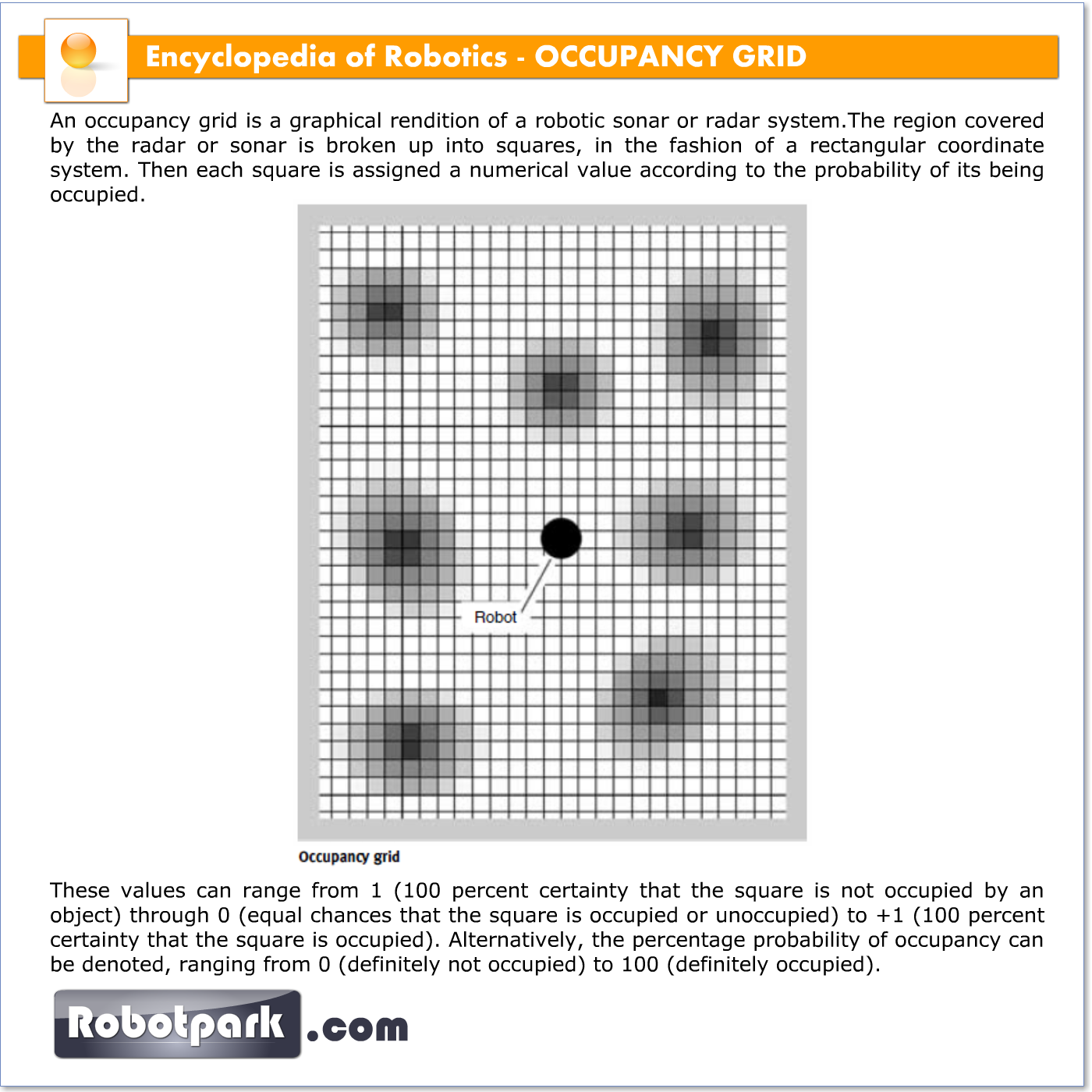# OCCUPANCY GRID – 21097

An occupancy grid is a graphical rendition of a robotic sonar or radar system.The region covered by the radar or sonar is broken up into squares, in the fashion of a rectangular coordinate system. Then each square is assigned a numerical value according to the probability of its being occupied. These values can range from 1 (100 percent certainty that the square is not occupied by an object) through 0 (equal chances that the square is occupied or unoccupied) to +1 (100 percent certainty that the square is occupied). Alternatively, the percentage probability of occupancy can be denoted, ranging from 0 (definitely not occupied) to 100 (definitely occupied).When rendered as a two-dimensional (2-D) figure, an occupancy grid consists of a set of squares or rectangles, each with a number inside, representing the probability that the square is occupied. However, an occupancy grid can be rendered using colors instead of numbers if less precision is demanded. This technique is often used in meteorological radar or satellite displays showing intensity of precipitation,wind speed, temperatures of cloud tops, or other variables. Probabilities of occupancy might be assigned as follows: violet = 0–17%; blue = 18–33%; green = 34–50%; yellow = 51–67%; orange = 68–83%; red = 84–100%.

Grayscale 2-D renditions of occupancy grids are possible.An example is shown in the illustration,which represents a hypothetical work environment in which several birds’ nests are located. This rendition has eight shades of gray. The darkest shades represent the highest probability that a bird is in a given sector at a given instant in time.White regions represent probabilities less than 1/8 (12.5 percent).

An occupancy grid can be rendered in three dimensions (3-D) by assigning the probabilities to values on an axis perpendicular to the plane of the grid itself. The regions of highest probability thus appear as “hills”or “mountains” while the regions of lowest probability appear as “valleys” or “canyons.”